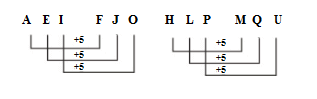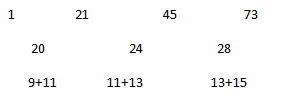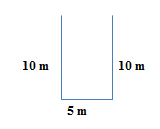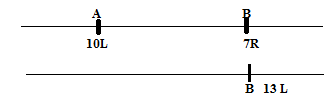# SSC CGL 2018 Practice Test Papers | Reasoning Ability (Day-28)

Dear Aspirants, Here we have given the Important SSC CGL Exam 2018 Practice Test Papers. Candidates those who are preparing for SSC CGL 2018 can practice these questions to get more confidence to Crack SSC CGL 2018 Examination.

[WpProQuiz 3079]

Click “Start Quiz” to attend these Questions and view Explanation

1) Select the related word/numbers/letters from the given alternatives.

AEI: FJO:: HLP:??

a) MQU

b) NPV

c) UIX

d) None of the above

2) Select the related word/numbers/letters from the given alternatives.

Frequency: Hertz :: Power:??

a) Newton

b) Watt

c) Joule

d) metre

3) Select the related word/numbers/letters from the given alternatives.

120:5::720??

a) 8

b)12

c)6

d) 9

4) Find the odd one.

a) CEG

b) GIK

c) ORT

d) WYA

5)  Find the odd one.

a) 1/3

b) 1/2

c)1/5

d)1/8

6) In a certain code language ‘SECRET’ is written as ‘DFTUFS’. Then, how will ‘RANGER’ be written in that code language ?

a) OBSSFH

b) OSBGFU

c) HFUEHL

d) URSBJI

7) A series is given, with one word missing. Choose the correct alternative from the given ones that will complete the series.

1, 21,45,73,?

a) 95

b) 105

c) 110

d) 200

8) If ‘L’ means ‘×’, ‘m’ means ‘÷’, ‘P’ means ‘+’, and ‘Q’ means ‘–’. Then find out the value of the equation ?

4 M 2 L 5 Q  7 P 1

a) 4

b) 6

c) 2

d) 9

9) A walks 10 m towards South and turns right and walks 5m and then turns left and walks 10 m. What is the distance between his Starting point and present position.

a) 5 m

b) 15 m

c) 10 m

d) 20 m

10) In a row Akash is 10th from the left and B is 7th from the right. If  both interchange their places, then B becomes 13th from the left. The number of persons in the row is :

a) 19

b) 20

c) 21

d) 22Unit of frequency is Hertz. Similarly, Unit of Power is watt

5!=120

6!=720

C+2=E;E+2=G

G+2=I;I+2=K

O+3=R;R+2=T

W+2=Y;Y+2=A

Except a) All others are rational number whereas 1/3 is an irrational number.

SECRET

i). Split the word into two halves and write in reverse order.

=> CES    TER.

ii). Code with next letter=> DFTUFS

R  A  N  G  E  R

i). Split the word into two halves and write in reverse order.

=>N  A  R    R  E  G

ii).Code with next letter=> OBRREG4/2 x 5-7+1 = 4